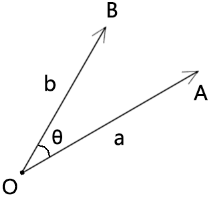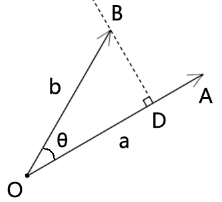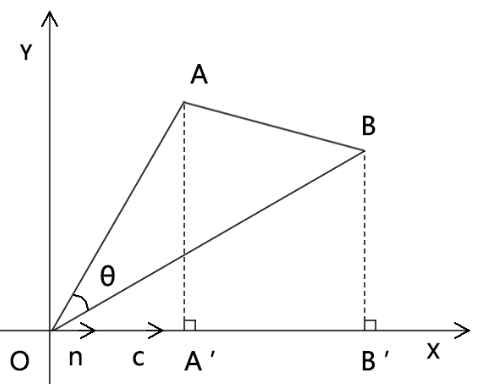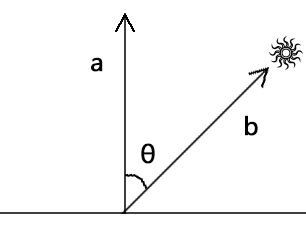## 前言

• 基于向量的长度和夹角求点积
• 基于向量的坐标点求点积

### 1-基本公式• 向量a的长度是|a|
• 向量b的长度是|b|
• 向量a和向量b的夹角是θ

``````a·b=|a|*|b|*cosθ
``````

### 2-向量在轴上的正射投影

``````a·b=|a|*|b|*cosθ
``````

``````cosθ=x/r
x=r*cosθ
``````

• |b|是不是可以作为斜边？
• |b|*cosθ 是不是一条临边？``````OD=|b|*cosθ
``````

• 正射投影的值是个实数，可正可负。
• 两个向量的夹角的值域是[0°,180°]
• 两个向量的夹角和点积的关系：
• 当θ=90° 时，b⊥a ，正射投影=0，点积=0
• 当θ=0° 时，b与a同向，正射投影=|b|，点积=|a|*|b|
• 当θ=180° 时，b与a共线，正射投影=-|b|，点积=-|a|*|b|
• 当θ∈[0°,90°) 时，正射投影>0，点积>0
• 当θ∈(90°,180°] 时，正射投影<0，点积<0
• a*b≤|a|*|b|，因为点积可正、可负、可零，|a|*|b|≥0

### 3-点积的运算律

#### 3-1-交换律

``````a·b=b·a
``````

``````a·b=|a|*|b|*cos<a,b>
b·a=|b|*|a|*cos<a,b>
``````

#### 3-2-分配率

``````(a+b)·c=a·c+b·c
``````• 向量OA
• 向量AB
• 向量OB
• 向量c
• 单位向量n
• 轴向量l
• 轴l、向量n和向量c共线
• 向量a和向量l的夹角为α
• AA'⊥l
• BB'⊥l

``````(OA+AB)·c=OA·c+AB·c
``````

``````AB·n=|OA|*|n|*cosα
``````

``````|n|=1
``````

``````OA·n=|OA|*|n|*cosα
OA·n=|OA|*1*cosα
OA·n=|OA|*cosα
OA·n=OA'
``````

``````OB·n=OB'
AB·n=A'B'
``````

``````OB=OA+AB
``````

``````(OA+AB)·n=OA·n+AB·n
``````

``````(OA+AB)·n*|c|=OA·n*|c|+AB·n*|c|
``````

``````c=n*|c|
``````

``````(OA+AB)·n*|c|=OA·n*|c|+AB·n*|c|
(OA+AB)·c=OA·c+AB·c
``````

#### 3-3-其它公式

``````(a+b)²=a²+2ab+b²
(a+b)·(a-b)=a²-b²

``````

### 1-基本公式

• 向量a和向量b所在的坐标系为二维直角坐标系
• 向量a的坐标点位是(a1,a2)
• 向量b的坐标点位是(b1,b2)

``````a·b=a1*b1+a2*b2
``````

``````a=a1*e1+a2*e2
b=b1*e1+b2*e2
``````

• a1,a2,b1,b2 都是实数
• e1,e2都是向量
• 实数乘以向量结果还是向量
• 向量和向量相加的结果还是向量

``````a·b=(a1*e1+a2*e2)*(b1*e1+b2*e2)
a·b=a1*b1*e2·e2+a1*b2*e1·e2+a2*b1*e1·e2+a2*b2*e2·e2
``````

``````e1·e1=|e1|*|e1|=1
e2·e2=|e2|*|e2|=1
``````

``````e1·e2=0
e2·e1=0
``````

``````a·b=a1*b1+a2*b2
``````

### 1-平行光下地面的受光强度• 地面的法线为单位向量a(ax,ay)
• 平行光的方向为单位向量b(bx,by)

``````a·b=|a|*|b|*cosθ
``````

``````cosθ=(a·b)/(|a|*|b|)
``````

``````|a|=sqrt(ax*ax+ay*ay)
|b|=sqrt(bx*bx+by*by)
``````

``````a·b=ax*bx+ay*by
``````

``````cosθ=(ax*bx+ay*by)/(sqrt(ax*ax+ay*ay)*sqrt(bx*bx+by*by))
``````

``````θ=0°
``````

``````cosθ=1
``````

``````θ=±90°
``````

``````cosθ=0
``````

## 发表评论

•• 匿名